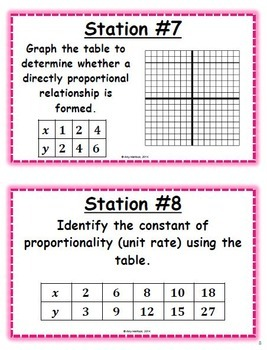# 7.RP Ratios and Proportional Relationships Task Cards6th - 8th
Subjects
Standards
Resource Type
Formats Included
• PDF
Pages
20 pages

#### Also included in

1. Your restless middle school math classes will LOVE task cards and station rotations. Let's face it - most students can't stand sitting in their desks all day. You want the cure for those tapping feet? Get them up and moving and watch their brains start moving too. Task cards make practice and re
\$39.99
\$66.23
Save \$26.24

### Description

Stations are the perfect activity for your restless and impatient students who like to get up and move around! The collaborative element makes them fun and engaging - a great change of pace from the typical warm up or test review!

Getting their bodies moving will often get their brains moving. Stations are an amazing warm up activity! With a variety of different questions, it’s definitely one of the most fun ways to review for a test.

Check out the comments and reviews - this resource is easy to use and students LOVE it!

7th Grade Common Core Math Standards from 7.RP.A.1, 7.RP.A.2.A, 7.RP.A.2.B, 7.RP.A.2.C, and 7.RP.A.2.D.

Analyze proportional relationships and use them to solve real-world and mathematical problems.

CCSS.MATH.CONTENT.7.RP.A.1

• Compute unit rates associated with ratios of fractions, including ratios of lengths, areas and other quantities measured in like or different units. For example, if a person walks 1/2 mile in each 1/4 hour, compute the unit rate as the complex fraction 1/2/1/4 miles per hour, equivalently 2 miles per hour.

CCSS.MATH.CONTENT.7.RP.A.2

• Recognize and represent proportional relationships between quantities.

CCSS.MATH.CONTENT.7.RP.A.2.A

• Decide whether two quantities are in a proportional relationship, e.g., by testing for equivalent ratios in a table or graphing on a coordinate plane and observing whether the graph is a straight line through the origin.

CCSS.MATH.CONTENT.7.RP.A.2.B

• Identify the constant of proportionality (unit rate) in tables, graphs, equations, diagrams, and verbal descriptions of proportional relationships.

CCSS.MATH.CONTENT.7.RP.A.2.C

• Represent proportional relationships by equations. For example, if total cost t is proportional to the number n of items purchased at a constant price p, the relationship between the total cost and the number of items can be expressed as t = pn.

CCSS.MATH.CONTENT.7.RP.A.2.D

• Explain what a point (x, y) on the graph of a proportional relationship means in terms of the situation, with special attention to the points (0, 0) and (1, r) where r is the unit rate.

HOW CAN YOU USE THIS ACTIVITY?

Cut out and laminate stations so you can use them every class period and every year!

I typically have students work in partners, but BOTH of them have to fill out the student information sheet, showing work. Students could also work individually. Working with more than one person gets too crowded, and some students skate by without participating at all.

Each group will start at a station. They will be given a certain amount of time to complete each task. At the end of the time, they will switch to the next station. Example: If a student starts at station 1, they will go to station 2. If they are at station 20, they will go to station 1.

There should never be more than two people at a station (unless you have more than 40 students…).

Encourage (or require) students to write down EVERY problem so that if they run out of time on one station, they can finish earlier problems at another station.

Give students a specific time to complete each task. (1-2 min) Use a timer that goes off to help students know when to switch stations. This way, when the timer goes off, students will just get up and move without direction. Determine the amount of time based on the skill set of each group. I give some classes more time than others if needed. If I start with 2 minutes and all of the students are finishing quickly, I will decrease the time as we go. Usually 2 minutes is too much!

I use this resource every year in the middle school math classroom. It can take up to a whole class period depending how much time is given to the students per station.

Before you buy, note: This product is part of my Complete Bundle of Math Stations and Task Cards

Related Products

Total Pages
20 pages
Included
Teaching Duration
55 minutes
Report this Resource to TpT
Reported resources will be reviewed by our team. Report this resource to let us know if this resource violates TpT’s content guidelines.

### Standards

to see state-specific standards (only available in the US).
Explain what a point (𝘹, 𝘺) on the graph of a proportional relationship means in terms of the situation, with special attention to the points (0, 0) and (1, 𝘳) where 𝘳 is the unit rate.
Represent proportional relationships by equations. For example, if total cost 𝘵 is proportional to the number 𝘯 of items purchased at a constant price 𝘱, the relationship between the total cost and the number of items can be expressed as 𝘵 = 𝘱𝘯.
Identify the constant of proportionality (unit rate) in tables, graphs, equations, diagrams, and verbal descriptions of proportional relationships.
Decide whether two quantities are in a proportional relationship, e.g., by testing for equivalent ratios in a table or graphing on a coordinate plane and observing whether the graph is a straight line through the origin.
Recognize and represent proportional relationships between quantities.